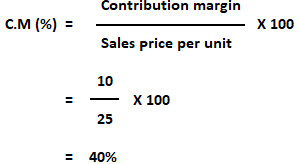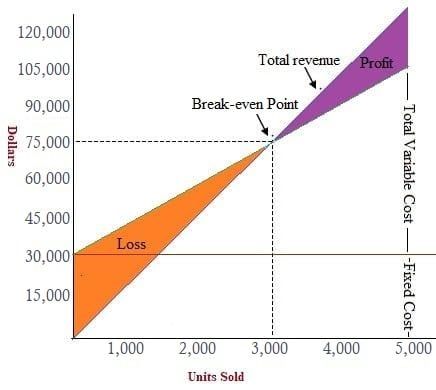Break-Even Point: Definition

The break-even point is the volume of activity at which a company’s total revenue equals the sum of all variable and fixed costs. The activity can be expressed in units or in dollar sales. The break-even point is the point at which there is no profit or loss.

At the break-even point, the total cost and selling price are equal, and the firm neither gains nor loses. The income of the business exactly equals its expenditure. This point is also known as the minimum point of production when total costs are recovered.

Break-Even Point: Explanation

It is possible to calculate the break-even point for an entire organization or for the specific projects, initiatives, or activities that an organization undertakes.

The basic objective of break-even point analysis is to ascertain the number of units of products that must be sold for the company to operate without loss. In other words, the no-profit-no-loss point is the break-even point.

Sales below the break-even point mean a loss, while any sales made above the break-even point lead to profits.

Formula For Break-Even Point

As you will be aware, profit can be calculated as sales revenues minus costs, where costs are either variable or fixed. That is to say,

Profit = Sales revenue – Variable costs – Fixed costs

where the sales revenue at break-even point = Fixed cost + Variable cost

This equation can be restated as follows:

Profit = (Unit sales price x Sales volume in units) – (Unit variable cost x Sales volume in units) – Fixed costs

Methods to Calculate Break-Even Point

This section provides an overview of the methods that can be applied to calculate the break-even point.

1. Algebraic/Equation Method

The following equation is helpful when finding the break-even point using the algebraic method:

SP = VC + FC

where

• SP = Sales price
• VC = Variable costs
• FC = Fixed costs

With this in mind, the following equation can be used to find the break-even point (o):

o = SP – VC – FC

Using the algebraic method, we can also identify the break-even point in unit or dollar terms, as illustrated below.

Example

Suppose that ABC Limited manufactures and sells a single product. The different costs per unit are as follows:

• SP per unit = \$25
• VC per unit = \$15
• Total FC = \$30,000

Required: Calculate the break-even point in units and dollars using the algebraic method.

Solution

The following formula can be used to calculate the sold number of units at the break-even point:

SP x Y = VC x Y + FC

where Y is the number of units sold to break-even. It follows that:

25 x Y = 15 x Y + 30,000

25Y = 15Y + 30,000

25Y – 15Y = 30,000

10Y = 30,000

Y = 30,000/10

Y = 3,000 units

Now, as we have calculated the break-even point in unit terms, we can easily compute the break-even point in dollars. To do this, we use the following equation:

BE point in dollars = BE point in units x SP

= 3,000 x 25

= \$75,000

2. Contribution Margin Method (or Unit Cost Basis)

It is also possible to compute the break-even point using the contribution margin method. Let’s consider the same figures for ABC Limited used in our example on the algebraic method.

CM = SP – VC

CM = \$25 – \$15

CM = \$10

Use the following formula to calculate the break-even point in sales units:

BE point = Fixed costs / CM per unit
= 30,000 / 10
= 3,000 units

Now, calculate the break-even point in  dollars using the following formula:

BE point (dollars) = Fixed cost / CM (expressed as a percentage of sales revenue)
= 30,000 / 40%*

BE point (dollars) = \$75,000

* C.M in percentage3. Budget Total Basis

Break-even point = Total fixed cost X (Sales / Contribution margin)

If the same cost data are available as in the example on the algebraic method, then the contribution is the same (i.e., \$16).

In addition, the break-even point would be 40,000 x (20/16) = 25,000 x 20 = \$50,000.

4. Graphical Presentation Method (Break-Even Chart or CVP Graph)

The break-even point or cost-volume-profit relationship can also be examined using graphs.Importance of Break-Even Point Analysis

Break-even point analysis can be applied to answer many important questions in business, including:

• What sales volume is required to produce the desired profits?
• What is the minimum level of sales needed to avoid losses?
• What effect will changes in fixed and variable costs have on profits?
• How will the change in sales mix in the context of a multiproduct firm affect profits?
• Which product is most profitable?
• What effect will a simultaneous change in price, cost, and volume have on profits?

True is a Certified Educator in Personal Finance (CEPF®), contributes to his financial education site, Finance Strategists, and has spoken to various financial communities such as the CFA Institute, as well as university students like his Alma mater, Biola University, where he received a bachelor of science in business and data analytics.

To learn more about True, visit his personal website, view his author profile on Amazon, his interview on CBS, or check out his speaker profile on the CFA Institute website.